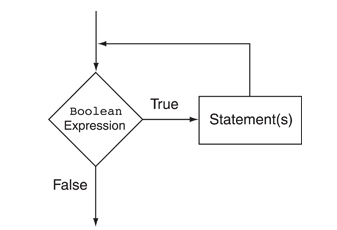# Chapter 6a - While Loops

##### While LoopThe BooleanExpression is tested, and if it is true, the Statement is executed. Then, the BooleanExpression is tested again. If it is true, the Statement is executed. This cycle repeats until the BooleanExpression is false.

## While Loop​

``import java.util.*;class Main {  public static void main(String[] args) {    int number = 0;    while (number<5)    {        System.out.println("Hello");        number++;    }      }}``
🧪 Try the code out~!### Practice​

``import java.util.Random;class Main {   public static void main(String args[]) {              int count = 10;        while (count >= 1)        {            System.out.println("Hello World");            System.out.println(count);            count--;        }   }}``

How many times will that code print `Hello World`?

### Exercise: Throwing random numbers​

##### Create a program that throws random numbers...
1. Create a program that generates random numbers from 0-9 (0 and 9 included) 5 times
2. Modify it so that it now generates 100 random numbers

You can create your program using the following template.

Hint

This is the code to print a random number from 0-100

🙋‍♀️ Expected Program (1) 5 random numbers from 0-9

🙋‍♀️ Expected Program (2) - 100 random numbers

## Infinite Loops​

##### Be careful and avoid creating an infinite loop• Infinite Loops

This code will be printing `Hello` forever

``import java.util.*;class Main {  public static void main(String[] args) {   int number = 1;    while (number <= 5)    {       System.out.println("Hello");    }      }}``
🧪 Try the code out~!

## Using While Loops to Validate Inputs​``import java.util.*;class Main {  public static void main(String[] args) {  int number;  // Create a Scanner object for keyboard input.  Scanner keyboard = new Scanner(System.in);    // Get a number from the user.  System.out.print("Enter a number in the range of 1 through 100: ");  number = keyboard.nextInt();    // Validate the input.  while (number < 1 || number > 100)  {     System.out.print("Invalid input. Enter a number in the range " +                      "of 1 through 100: ");     number = keyboard.nextInt();  }      }}``
🧪 Try the code out~!

### Exercise: Generate Random numbers until...​

##### Exercise
• Create a program that generates random numbers from 0-9 until it generates number 5
• You can use the following template to start your code:

🙋‍♀️ Expected Program